International
Tables for
Crystallography
Volume B
Reciprocal space
Edited by U. Shmueli

International Tables for Crystallography (2006). Vol. B. ch. 4.5, pp. 467-469   | 1 | 2 |

## Section 4.5.2.3. Diffraction by helical structures

R. P. Millanea*

#### 4.5.2.3. Diffraction by helical structures

| top | pdf |

Molecules or assemblies studied by fibre diffraction are usually made up of a large number of identical, or nearly identical, residues, or subunits, that in an oriented specimen are distributed along an axis; this leads naturally to helical symmetry. Since a periodic structure with no helix symmetry can be treated as a onefold helix, the assumption of helix symmetry is not restrictive.

#### 4.5.2.3.1. Helix symmetry

| top | pdf |

The presence of a unique axis about which there is rotational disorder means that it is convenient to use cylindrical polar coordinate systems in fibre diffraction. We denote by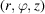a cylindrical polar coordinate system in real space, in which the z axis is parallel to the molecular axes. The molecule is said to have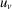helix symmetry, where u and v are integers, if the electron density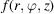satisfies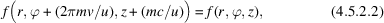where m is any integer. The constant c is the period along the z direction, which is referred to variously as the molecular repeat distance, the crystallographic repeat, or the c repeat. The helix pitch P is equal to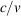. Helix symmetry is easily interpreted as follows. There are u subunits, or helix repeat units, in one c repeat of the molecule. The helix repeat units are repeated by integral rotations of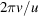about, and translations of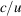along, the molecular (or helix) axis. The helix repeat units may therefore be referenced to a helical lattice that consists of points at a fixed radius, with relative rotations and translations as described above. These points lie on a helix of pitch P, there are v turns (or pitch-lengths) of the helix in one c repeat, and there are u helical lattice points in one c repeat. Ahelix is said to have `u residues in v turns'.

Since the electron density is periodic in ϕ and z, it can be decomposed into a Fourier series as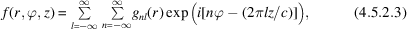where the coefficients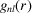are given by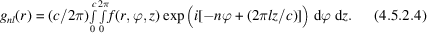Assume now that the electron density has helical symmetry. Denote by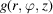the electron density in the region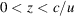; the electron density being zero outside this region, i.e.is the electron density of a single helix repeat unit. It follows that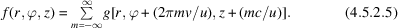Substituting equation (4.5.2.5)into equation (4.5.2.4)shows thatvanishes unless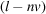is a multiple of u, i.e. unless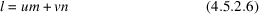for any integer m. Equation (4.5.2.6)is called the helix selection rule. The electron density in the helix repeat unit is therefore given by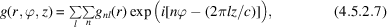where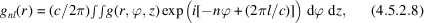and where in equation (4.5.2.7)(and in the remainder of this section) the sum over l is over all integers, the sum over n is over all integers satisfying the helix selection rule and the integral in equation (4.5.2.8)is over one helix repeat unit. The effect of helix symmetry, therefore, is to restrict the number of Fourier coefficientsrequired to represent the electron density to those whose index n satisfies the selection rule. Note that the selection rule is usually derived using a rather more complicated argument by considering the convolution of the Fourier transform of a continuous filamentary helix with a set of planes in reciprocal space (Cochran et al., 1952). The approach described above, which follows that of Millane (1991), is much more straightforward.

#### 4.5.2.3.2. Diffraction by helical structures

| top | pdf |

Denote by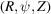a cylindrical polar coordinate system in reciprocal space (with the Z and z axes parallel), and by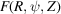the Fourier transform of. Sinceis periodic in z with period c, its Fourier transform is nonzero only on the layer planes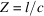where l is an integer. Denote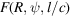by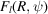; using the cylindrical form of the Fourier transform shows that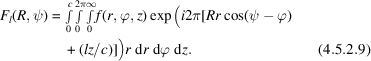It is convenient to rewrite equation (4.5.2.9)making use of the Fourier decomposition described in Section 4.5.2.3.1, since this allows utilization of the helix selection rule. The Fourier–Bessel structure factors (Klug et al., 1958),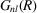, are defined as the Hankel transform of the Fourier coefficients, i.e.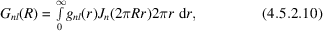and the inverse transform is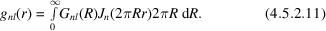Using equations (4.5.2.7)and (4.5.2.11)shows that equation (4.5.2.9)can be written as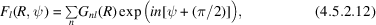where, as usual, the sum is over only those values of n that satisfy the helix selection rule. Using equations (4.5.2.8)and (4.5.2.10)shows that the Fourier–Bessel structure factors may be written in terms of the atomic coordinates as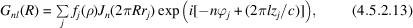where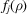is the (spherically symmetric) atomic scattering factor (usually including an isotropic temperature factor) of the jth atom and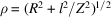is the spherical radius in reciprocal space. Equations (4.5.2.12)and (4.5.2.13)allow the complex diffracted amplitudes for a helical molecule to be calculated from the atomic coordinates, and are analogous to expressions for the structure factors in conventional crystallography.

The significance of the selection rule is now more apparent. On a particular layer plane l, not all Fourier–Bessel structure factorscontribute; only those whose Bessel order n satisfies the selection rule for that value of l contribute. Since any molecule has a maximum radius, denoted here by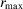, and since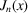is small for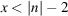and diffraction data are measured out to only a finite value of R, reference to equation (4.5.2.10)[or equation (4.5.2.13)] shows that there is a maximum Bessel order that contributes significant value to equation (4.5.2.12)(Crowther et al., 1970; Makowski, 1982), so that the infinite sum over n in equation (4.5.2.12)can be replaced by a finite sum. On each layer plane there is also a minimum value of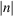, denoted by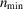, that satisfies the helix selection rule, so that the region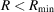is devoid of diffracted amplitude where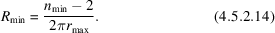The selection rule therefore results in a region around the Z axis of reciprocal space that is devoid of diffraction, the shape of the region depending on the helix symmetry.

#### 4.5.2.3.3. Approximate helix symmetry

| top | pdf |

In some cases the nature of the subunits and their interactions results in a structure that is not exactly periodic. Consider a helical structure with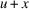subunits in v turns, where x is a small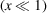real number; i.e. the structure has approximate, but not exact,helix symmetry. Since the molecule has an approximate repeat distance c, only those layer planes close to those atshow significant diffraction. Denoting by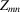the Z coordinate of the nth Bessel order and its associated value of m, and using the selection rule shows that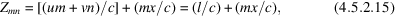so that the positions of the Bessel orders are shifted by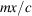from their positions if the helix symmetry is exactly. At moderate resolution m is small so the shift is small. Hence Bessel orders that would have been coincident on a particular layer plane are now separated in reciprocal space. This is referred to as layer-plane splitting and was first observed in fibre diffraction patterns from tobacco mosaic virus (TMV) (Franklin & Klug, 1955). Splitting can be used to advantage in structure determination (Section 4.5.2.6.6).

As an example, TMV has approximately 493 helix symmetry with a c repeat of 69 Å. However, close inspection of diffraction patterns from TMV shows that there are actually about 49.02 subunits in three turns (Stubbs & Makowski, 1982). The virus is therefore more accurately described as a 2451150 helix with a c repeat of 3450 Å. The layer lines corresponding to this larger repeat distance are not observed, but the effects of layer-plane splitting are detectable (Stubbs & Makowski, 1982).

### ReferencesCochran, W., Crick, F. H. C. & Vand, V. (1952). The structure of synthetic polypeptides. I. The transform of atoms on a helix. Acta Cryst. 5, 581–586.Google ScholarCrowther, R. A., DeRosier, D. J. & Klug, A. (1970). The reconstruction of a three-dimensional structure from projections and its application to electron microscopy. Proc. R. Soc. London Ser. A, 317, 319–344.Google ScholarFranklin, R. E. & Klug, A. (1955). The splitting of layer lines in X-ray fibre diagrams of helical structures: application to tobacco mosaic virus. Acta Cryst. 8, 777–780.Google ScholarKlug, A., Crick, F. H. C. & Wyckoff, H. W. (1958). Diffraction from helical structures. Acta Cryst. 11, 199–213.Google ScholarMakowski, L. (1982). The use of continuous diffraction data as a phase constraint. II. Application to fibre diffraction data. J. Appl. Cryst. 15, 546–557.Google ScholarMillane, R. P. (1991). An alternative approach to helical diffraction. Acta Cryst. A47, 449–451.Google ScholarStubbs, G. J. & Makowski, L. (1982). Coordinated use of isomorphous replacement and layer-line splitting in the phasing of fibre diffraction data. Acta Cryst. A38, 417–425.Google Scholar